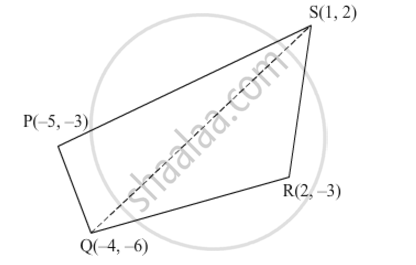# If P(–5, –3), Q(–4, –6), R(2, –3) and S(1, 2) are the vertices of a quadrilateral PQRS, find its area. - Mathematics

If P(–5, –3), Q(–4, –6), R(2, –3) and S(1, 2) are the vertices of a quadrilateral PQRS, find its area.

#### SolutionThe vertices of the quadrilateral PQRS are P(−5, −3), Q(−4, −6), R(2, −3) and S(1, 2).

Join QS to form two triangles, namely, ΔPQS and ΔRSQ.

Area of quadrilateral PQRS = Area of ΔPQS + Area of ΔRSQ

We know

Area of triangle having vertices (x1, y1), (x2, y2) and (x3, y3) = 1/2[x1(y2y3)+x2(y3y1)+x3(y1y2)]

Now,

Area of PQS 1/2[5(62)+(4)(2+3)+1(3+6)]

=1/2[5(8)+(4)(5)+1(3)]

=1/2(4020+3)

=23/2square units

Area of RSQ1/2[2(2+6)+1(6+3)+(4)(32)]

=1/2[2(8)+1(3)+(4)(5)]

=1/2(163+20)

=33/2 square units

Area of quadrilateral PQRS = Area of ΔPQS + Area of ΔRSQ

=23/2+33/2

=56/2

=28 square units

Thus, the area of quadrilateral PQRS is 28 square units.

Concept: Area of a Triangle
Is there an error in this question or solution?
Chapter 6: Co-Ordinate Geometry - Exercise 6.5 [Page 54]

#### APPEARS IN

RD Sharma Class 10 Maths
Chapter 6 Co-Ordinate Geometry
Exercise 6.5 | Q 9 | Page 54
Share